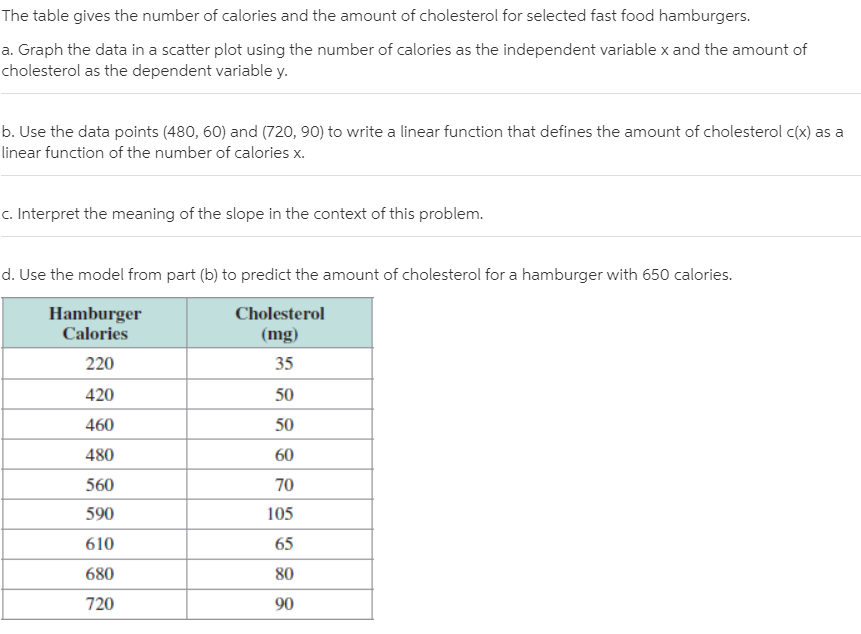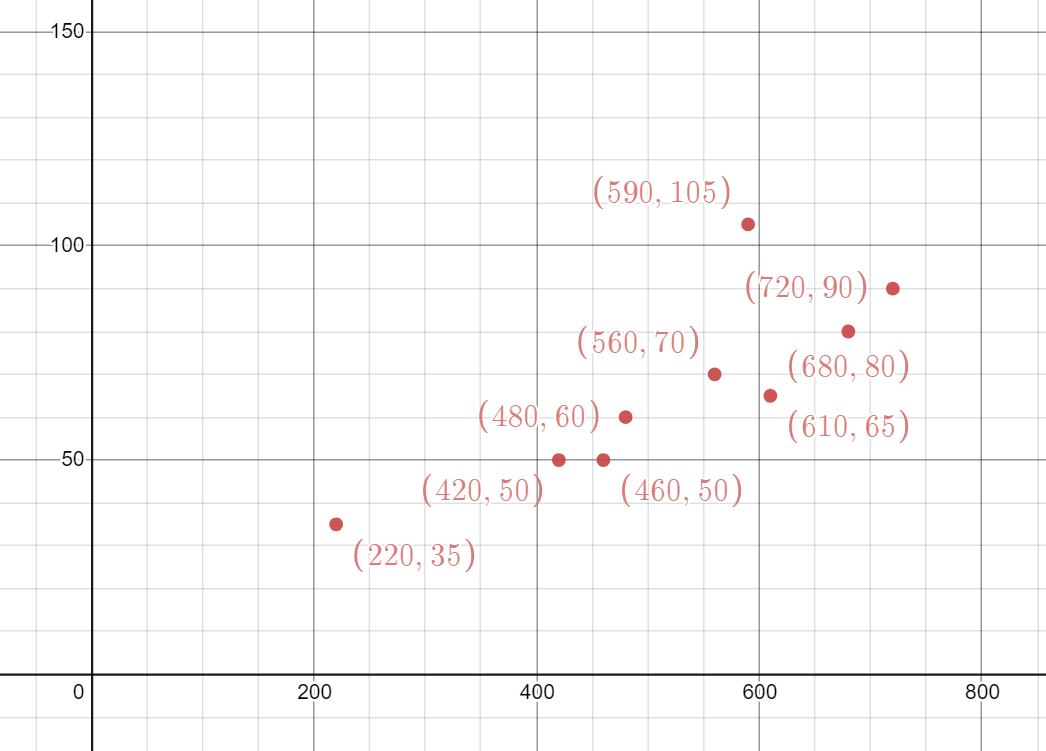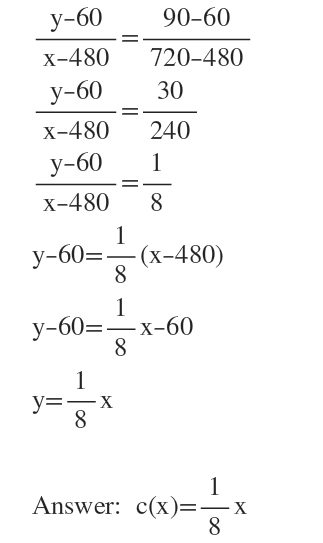# The table gives the number of calories and the amount of cholesterol for selected fast food hamburgers.a. Graph the data in a scatter plot using the number of calories as the independent variable x and the amount ofcholesterol as the dependent variable y.b. Use the data points (480, 60) and (720, 90) to write a linear function that defines the amount of cholesterol c(x) as alinear function of the number of calories x.c. Interpret the meaning of the slope in the context of this problem.d. Use the model from part (b) to predict the amount of cholesterol for a hamburger with 650 calories.HamburgerCaloriesCholesterol(mg)2203542050460504806056070590105610656808072090

Question
1 viewshelp_outlineImage TranscriptioncloseThe table gives the number of calories and the amount of cholesterol for selected fast food hamburgers. a. Graph the data in a scatter plot using the number of calories as the independent variable x and the amount of cholesterol as the dependent variable y. b. Use the data points (480, 60) and (720, 90) to write a linear function that defines the amount of cholesterol c(x) as a linear function of the number of calories x. c. Interpret the meaning of the slope in the context of this problem. d. Use the model from part (b) to predict the amount of cholesterol for a hamburger with 650 calories. Hamburger Calories Cholesterol (mg) 220 35 420 50 460 50 480 60 560 70 590 105 610 65 680 80 720 90 fullscreen
check_circle

Step 1

We graph the points as scatter plot.Step 2

b) We will use the two-point formula to write the equation....

### Want to see the full answer?

See Solution

#### Want to see this answer and more?

Solutions are written by subject experts who are available 24/7. Questions are typically answered within 1 hour.*

See Solution
*Response times may vary by subject and question.
Tagged in